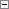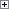You can insert comments about your C/AL code or comment out parts of your code to prevent execution. The following are the two ways to insert comments in C/AL:

• Use "//" to insert a single line comment. When the "//" symbol is encountered in your code, the rest of the line is interpreted as a comment.
• Use "{" and "}" to mark the start and end, respectively, of a block of comments.

You can nest any number of comments. In such cases, the comment runs from the first comment start to the last comment end.

Example 1Copy Code
{
This is a sample comment which is ignored by the C/AL compiler
}

Example 2Copy Code
// This is also a sample comment which is ignored by the C/AL compiler

Example 3Copy Code
{ This comment { is partly inside } another comment }

Example 4

This example shows what you should not do.Copy Code
A := 34;
B := 56;      {******************
C := 345;      * Don’t do this! *
D := 781;      ******************}

Because the comment is to the right of the C/AL statements, the system assumes that the third and fourth lines are part of the comment. That is, only A and B are assigned values, while C and D are not. Instead you should use single line comments.Copy Code
A := 34;
B := 56;       //*******************
C := 345;      //* Do it this way! *
D := 781;      //*******************